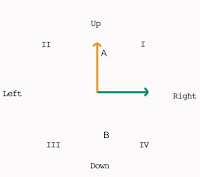## Tuesday, February 28, 2017

### The Direction of Forces in Two DimensionsWhen adding forces in two dimensions, it is easy to find the magnitude of the resultant force using the Pythagorean Theorem. The resultant is the hypotenuse of a triangle made by the two forces.

It is also easy to find the direction of the two forces. First, look at the image to the right.

Force A is up and Force B is at a right angle to it (also known as being orthogonal).

Because of the nature of vectors, and because forces are vectors, the image can be redrawn so that the tail of one force originates at the head of the other.

The result of that is to the left, below.

When viewed in this way, it is easy to see that the resultant force is the hypothesis of the two sides, represented by the blue line.The angle, which is the direction of the force is represented as ø and the sides are labeled O for opposite the angle, and A for adjacent to the angle.

So the green side is adjacent to the angle (it touches) and the gold side is opposite the angle (it does not touch).

Now, through the magic of trigonometry, we can use the fact that we have a right angle to calculate the angle.

The end product of all the math is that you need only divide the magnitude of opposite by the magnitude of the adjacent, and then hit the equal sign, and then hit one more button on your calculator.

Since it is soooo easy, here's the math!

By definition the tangent is a number. Because of the magic of math, for any triangle with one right angle, the sides are always in the same proportion, and are represented as a decimal, such as .7832 or .3231. There used to be books with pages and pages of tables so that the angle could be looked up for any given decimal. (IKR?)

So, the way that gets written is this:

tan(ø) = O/A

Wow.

So if you divide O by A you get a decimal decimal number. Then you go look that up and find the angle. Except, no.

There is a button on your calculator for that!

So, more math. We can solve for ø by doing the division, and then doing the un-tangent process. The actual name is the inverse tangent or (so to be a fuzz more confusing) arctangent.

So, look at this:

Okay, now for the calculator!

To find the direction of a resultant force, resolve all the forces into two orthogonal forces. Then draw out the triangles. Draw the hypotenuse. Then, you are ready.

First, divide the opposite by the adjacent and hit the equal button.

Now the fun! Find the inverse tangent button! Good luck with that! This is what it looks like on the laptop calculator (You MUST hit the "2nd" button first!):

On the TI-30, you also have to hit the "2nd" button first:

So, after you find the button, you are pretty much there.

Example:

Force A has a magnitude of 5 N Up
Force B has a magnitude of 7 N Right

Find the direction in degrees of the resultant force.

tan(ø) = 5/7

Divide 5 by 7 = .71428

Push the inverse tan button. The angle is 35.54 degrees.

Done.

The method for finding the direction of the resultant force when two orthogonal forces is relatively easy. Once the button is found, it takes only two steps on the calculator. While the theory and foundations come from trigonometry, it is not necessary to fully understand the math to use it.